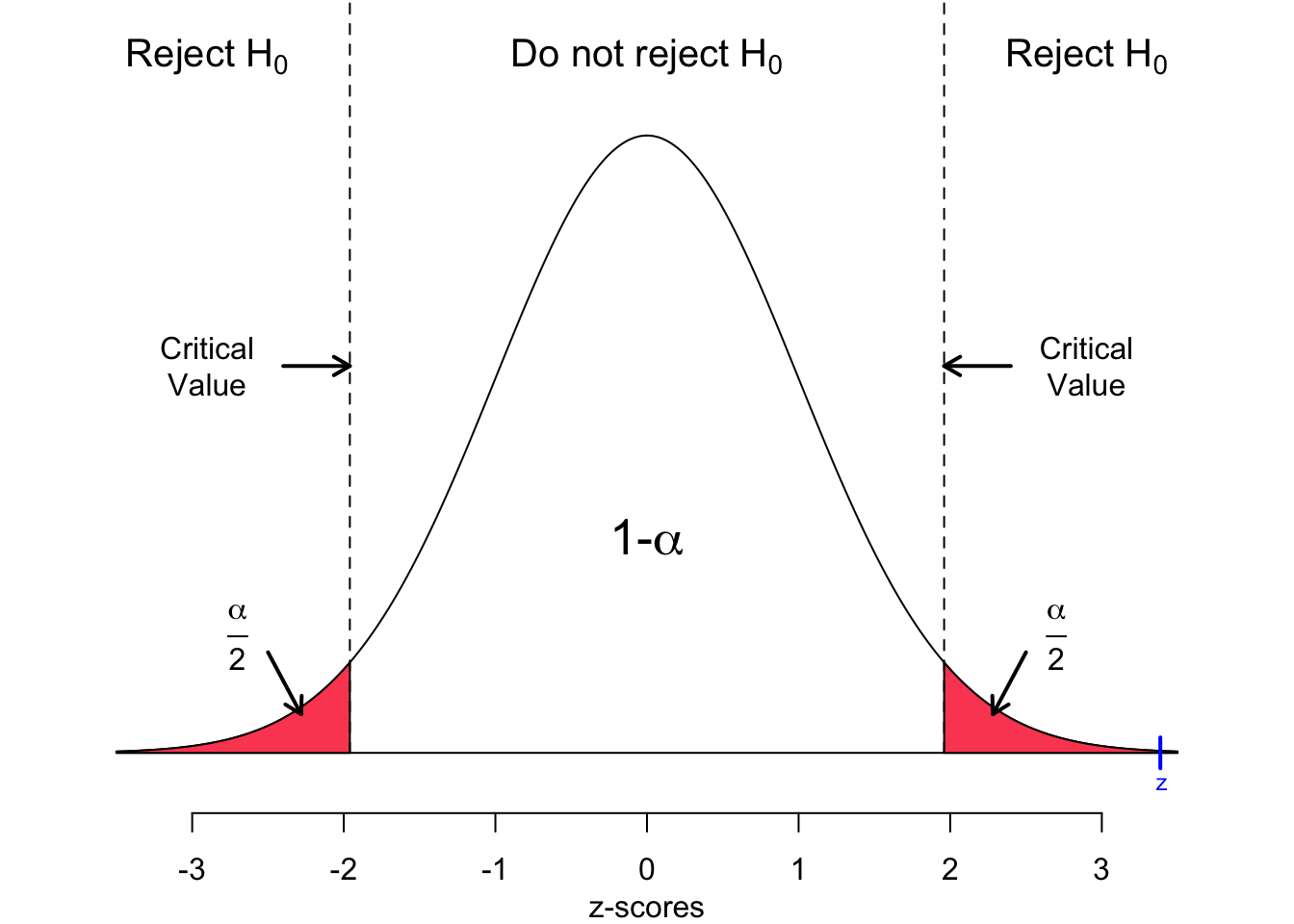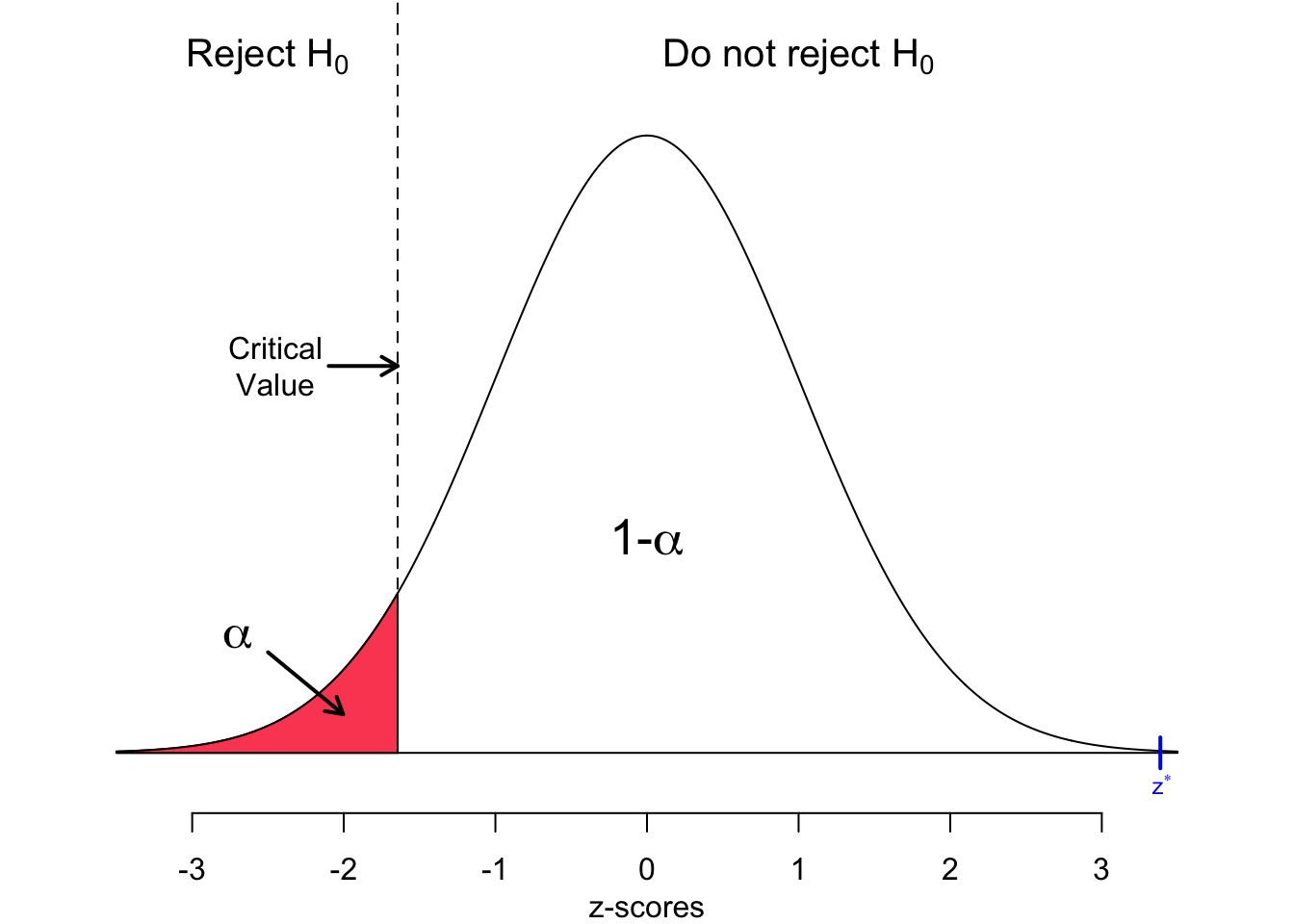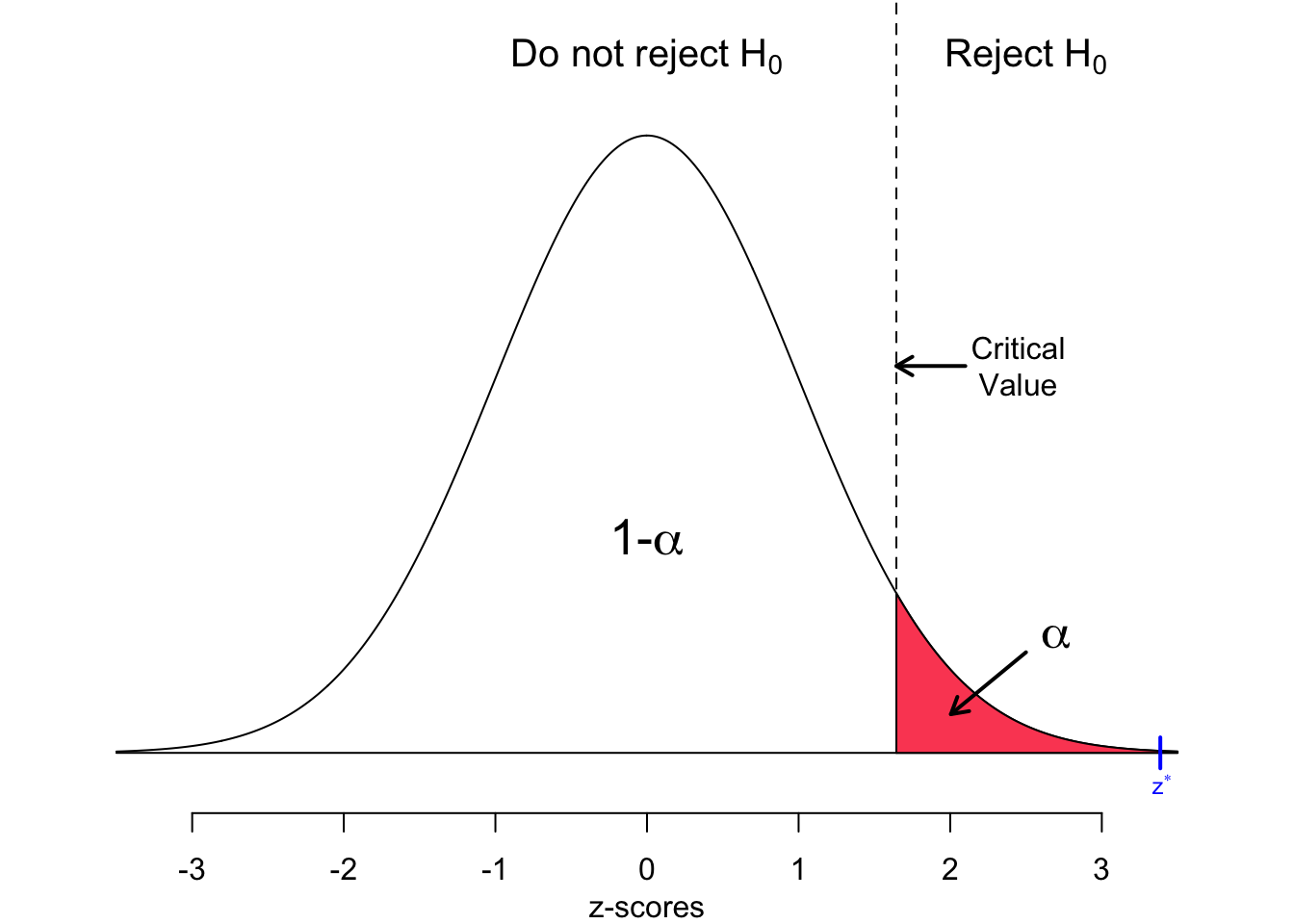If the population standard deviation ($$\sigma$$) is known, a hypothesis test performed for one population mean is called one-mean $$z$$-test or simply $$z$$-test.

A $$z$$-test is a hypothesis test for testing a population mean, $$\mu$$, against a supposed population mean, $$\mu_0$$. The $$z$$-test assumes normally distributed variables or a large sample size; then the central limit theorem guarantees a normally distributed sampling distribution. In addition, $$\sigma$$, the standard deviation of the population must be known. In real life applications this assumption is almost never fulfilled, and thus, the $$z$$-test is rarely applied. However, it is the simplest hypothesis test and thus, a good subject to start with.

To perform the $$z$$-test we follow the step wise procedure, as shown in the table below. First, we showcase the critical value approach, then, in a second step, we repeat the analysis for the p-value approach.

$\begin{array}{l} \hline \ \text{Step 1} & \text{State the null hypothesis } H_0 \text{ and alternative hypothesis } H_A \text{.}\\ \ \text{Step 2} & \text{Decide on the significance level, } \alpha\text{.} \\ \ \text{Step 3} & \text{Compute the value of the test statistic.} \\ \ \text{Step 4a} & \text{Critical value approach: Determine the critical value.} \\ \ \text{Step 4b} &\text{P-value approach: Determine the p-value.} \\ \ \text{Step 5a} & \text{Critical value approach: If the value of the test statistic falls in the rejection region, reject} H_0 \text{; otherwise, do not reject} H_0 \text{.} \\ \ \text{Step 5b} & \text{P-value approach: If } p \le \alpha \text{, reject }H_0 \text{; otherwise, do not reject } H_0 \text{.} \\ \ \text{Step 6} &\text{Interpret the result of the hypothesis test.} \\ \hline \end{array}$

#### One-mean $$z$$-test: An example

In this section we work with the students data set. You may download the students.csv file here. Import the data set and assign a proper name to it.

students <- read.csv("https://userpage.fu-berlin.de/soga/200/2010_data_sets/students.csv")

The students data set consists of 8239 rows, each of them representing a particular student, and 16 columns, each of them corresponding to a variable/feature related to that particular student. These self-explaining variables are: stud.id, name, gender, age, height, weight, religion, nc.score, semester, major, minor, score1, score2, online.tutorial, graduated, salary.

In order to showcase hypothesis testing we examine the average weight of students and compare it to the average weight of Europeans adults. Walpole et al. (2012) published data on the average body mass (kg) per region, including Europe. They report the mean the average body mass for the European adult population to be 70.8 kg. We set $$\mu_0$$, the population mean accordingly, $$\mu_0 = 70.8$$. Unfortunately, owing to the methodological approach of Walpole et al. (2012) they did not provide a standard deviation ($$\sigma$$) of the weights of European adults. For demonstration purposes we assume that the weight data given in the students data set is a good approximation for the population of interest. Thus, we set $$\sigma$$ to the standard deviation of the weight variable in the students data set.

mu0 <- 70.8
sigma <- sd(students$weight) sigma ##  8.635162 Further, we take one random samples with a sample size of $$n=14$$. The sample consists of the weights in kg of 14 randomly picked students from the students data set. Finally, we calculate the sample mean ($$\bar x$$), which is our sample statistic of intertest. The sample statistic is assigned to the variable x.bar. n <- 14 # random sampling x.weight <- sample(x = students$weight, size = n)
# calculate sample mean
x.bar <- mean(x.weight)
x.bar
##  78.61429

#### Hypothesis testing: The critical value approach

Step 1: State the null hypothesis, $$H_0$$, and alternative hypothesis, $$H_A$$.

The null hypothesis states that the average weight of students ($$\mu$$) equals the average weight of European adults of 70.8 kg ($$\mu_0$$) as reported by Walpole et al. (2012). In other words, there is no difference in the mean weight of students and the mean weight of European adults.

$H_0: \quad \mu = 70.8$ For the purpose of illustration we test three alternative hypothesis.

Alternative hypothesis 1: The average weight of students does not equal the average weight of European adults. In other words there is a difference in the mean weight of students and the mean weight of European adults. $H_{A_1}: \quad \mu \ne 70.8$

Alternative hypothesis 2: The average weight of students is less than the average weight of European adults. $H_{A_2}: \quad \mu < 70.8$

Alternative hypothesis 3: The average weight of students is higher than the average weight of European adults. $H_{A_3}: \quad \mu > 70.8$

Step 2: Decide on the significance level, $$\alpha$$. $\alpha = 0.05$

alpha <- 0.05

Step 3: Compute the value of the test statistic.

The following equation is applied to calculate the test statistic $$z$$.

$z = \frac{\bar x-\mu_0}{\sigma/\sqrt{n}}$

• Compute the value of the test statistic

$z = \frac{\bar x-\mu_0}{\sigma/\sqrt{n}} = \frac{78.61-70.8}{8.64/ \sqrt{14}} \approx 3.39$

z <- (x.bar-mu0) / sigma*sqrt(n)
z
##  3.385968

Step 4a: Determine the critical value.

In order to calculate the critical value we apply the qnorm() function in R. Recall, that we test for three alternative hypothesis ($$H_{A_1}$$, $$H_{A_2}$$, and $$H_{A_3}$$), thus, we have to calculate three critical values as well ($$z_{A_1} = \pm z_{\alpha/2}$$, $$z_{A_2} = -z_\alpha$$, and $$z_{A_3} = +z_\alpha$$).

z.HA.1 <- qnorm(1 - alpha/2)
z.HA.2 <- qnorm(1 - alpha, lower.tail = FALSE)
z.HA.3 <- qnorm(1 - alpha)

The critical values are $$z_{A_1} \approx \pm 1.96$$, $$z_{A_2} \approx -1.64$$, and $$z_{A_3} \approx 1.64$$.

Step 5a: If the value of the test statistic falls in the rejection region, reject $$H_0$$; otherwise, do not reject $$H_0$$.

The value of the test statistic found in Step 3 is $$z \approx 3.39$$. Recall, that we are investigating three alternative hypothesis ($$H_{A_1}$$,$$H_{A_2}$$, and $$H_{A_3}$$). Thus, we evaluate the rejection region for each particular hypothesis.

• Alternative hypothesis $$H_{A_1}:\quad \mu \ne 70.8$$

Recall the critical values for $$H_{A_1}$$. $z_{A_1} = \pm z_{\alpha/2} = \pm 1.96$

Does the test statistic ($$z \approx 3.39$$) fall in the rejection region? Be aware that the test is two tailed, thus, we evaluate the upper and the lower limit.

• Upper limit

$3.39 > 1.96$

# upper limit
# Reject?
print(z > abs(z.HA.1))
##  TRUE
• Lower limit

$3.39 < -1.96$

#lower limit
# Reject?
print(z < -abs(z.HA.1))
##  FALSEBased on the numerical and graphical evaluation the value does fall in the rejection region, so we reject $$H_0$$. The test results are statistically significant at the 5% level.

• Alternative hypothesis $$H_{A_2}:\quad \mu < 70.8$$

Recall the critical value for $$H_{A_2}$$. $z_{A_2} = -z_{\alpha} = -1.64$

Does the test statistic ($$z \approx 3.39$$) fall in the rejection region?

$3.39 < -1.64$

# Reject?
print(z < z.HA.2)
##  FALSEBased on the numerical and graphical evaluation the value does not fall in the rejection region, so we do not reject $$H_0$$. The test results are statistically significant at the 5% level.

• Alternative hypothesis $$H_{A_3}:\quad \mu > 70.8$$

Recall the critical value for $$H_{A_3}$$. $z_{A_3} = +z_{\alpha} = 1.64$

Does the test statistic ($$z \approx 3.39$$) fall in the rejection region?

$3.39 > 1.64$

# Reject?
print(z > z.HA.3)
##  TRUEBased on the numerical and graphical evaluation the value does fall in the rejection region, so we reject $$H_0$$. The test results are statistically significant at the 5% level.

Step 6: Interpret the result of the hypothesis test.

• Alternative hypothesis $$H_{A_1}:\quad \mu \ne 70.8$$

At the 5% significance level, the data provides sufficient evidence to conclude that the average weight of students differs from the average weight European adults.

• Alternative hypothesis $$H_{A_2}:\quad \mu < 70.8$$

At the 5% significance level, the data does not provide sufficient evidence to conclude that the average weight of students is less than the average weight of European adults.

• Alternative hypothesis $$H_{A_3}:\quad \mu > 70.8$$

At the 5% significance level, the data provides sufficient evidence to conclude that the average weight of students is higher than average weight of European adults.

#### Hypothesis testing: The p-value approach

Step 1: State the null hypothesis, $$H_0$$, and alternative hypothesis, $$H_A$$.

The null hypothesis states that the average weight of students ($$\mu$$) equals the average weight of European adults of 70.8 kg ($$\mu_0$$) as reported by Walpole et al. (2012). In other words, there is no difference in the mean weight of students and the mean weight of European adults.

$H_0: \quad \mu = 70.8$ For the purpose of illustration we test three alternative hypothesis.

Alternative hypothesis 1: The average weight of students does not equal the average weight of European adults. In other words there is a difference in the mean weight of students and the mean weight of European adults. $H_{A_1}: \quad \mu \ne 70.8$

Alternative hypothesis 2: The average weight of students is less than the average weight of European adults. $H_{A_2}: \quad \mu < 70.8$

Alternative hypothesis 3: The average weight of students is higher than the average weight of European adults. $H_{A_3}: \quad \mu > 70.8$

Step 2: Decide on the significance level, $$\alpha$$. $\alpha = 0.05$

alpha <- 0.05

Step 3: Compute the value of the test statistic.

The following equation is applied to calculate the test statistic $$z$$.

$z = \frac{\bar x-\mu_0}{\sigma/\sqrt{n}}$

• Compute the value of the test statistic

$z = \frac{\bar x-\mu}{\sigma/\sqrt{n}} = \frac{78.61-70.8}{8.64/ \sqrt{14}} \approx 3.39$

z <- (x.bar-mu0)/sigma*sqrt(n)
z
##  3.385968

Step 4b: Determine the p-value.

In order to calculate the p-value we apply the pnorm() function in R. Recall, that we test for three alternative hypothesis ($$H_{A_1}$$, $$H_{A_2}$$, and $$H_{A_3}$$), thus we calculate three p-values as well $$(P(z_{A_1})$$, $$P(z_{A_2})$$, and $$P(z_{A_3}))$$.

• Alternative hypothesis $$H_{A_1}:\quad \mu \ne 70.8$$
#the probability of observing any z-value greater or smaller in magnitude given the null hypothesis is true
upper <- pnorm(abs(z), lower.tail = FALSE)
lower <- pnorm(-abs(z), lower.tail = TRUE)
p.z.1 <- upper + lower
p.z.1 
##  0.0007092769

From step 3, the value of the test statistic is $$z \approx 3.39$$. The test is two-sided, so the p-value is the probability of observing a value $$z$$ of 3.39 or greater in magnitude, or a value $$z$$ of -3.39 or lower in magnitude. That probability equals the colored area in the figure below, which is 2 x 0 or 0.001. Thus, $$p \approx 0.001$$.